# MISSION BANKING 2023 Quantitative Aptitude Quiz- 13

## MISSION BANKING 2023 Quantitative Aptitude Quiz

Quantitative Aptitude is the most significant part of almost all competitive exams. Candidates appear to be having difficulty with Quantitative Aptitude Questions, so they need to practice more. Even if the portion is difficult, if you practice enough questions and learn the basic concepts, you can surely do well. In this article, we have come up with the MISSION BANKING 2023 Quantitative Aptitude Quiz to enhance your preparation. This MISSION BANKING 2023 Quantitative Aptitude Quiz will assist you to cope with the tough competition. This MISSION BANKING 2023 Quantitative Aptitude Quiz is available to you at no cost. Through this MISSION BANKING 2023 Quantitative Aptitude Quiz, you will learn formulas and short tricks to solve the Quantitative Aptitude Questions. Candidates must solve this MISSION BANKING 2023 Quantitative Aptitude Quiz to improve their exam.(a) if x<y

(b) if x>y

(c) if x≤y

(d) if x≥y

(e) if x=y or No relation can be established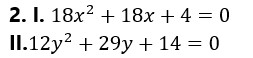(a) if x<y

(b) if x>y

(c) if x≤y

(d) if x≥y

(e) if x=y or No relation can be established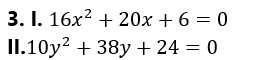(a) if x<y

(b) if x>y

(c) if x≤y

(d) if x≥y

(e) if x=y or No relation can be established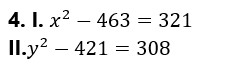(a) if x<y

(b) if x>y

(c) if x≤y

(d) if x≥y

(e) if x=y or No relation can be established(a) if x<y

(b) if x>y

(c) if x≤y

(d) if x≥y

(e) if x=y or No relation can be established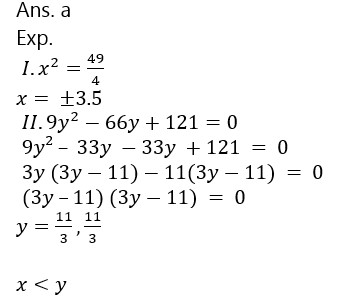1. Two jar A and B contain a mixture of two liquids, X and Y in the ratio of 2 : 3 and 4 : 5 respectively. If mixture from jar A and B are mixed in the ratio of 1 : 2 then, find the ratio of X and Y in final mixture.

(a) 70:51

(b) 58:77

(c) 61:77

(d) 77:58

(e) 62:61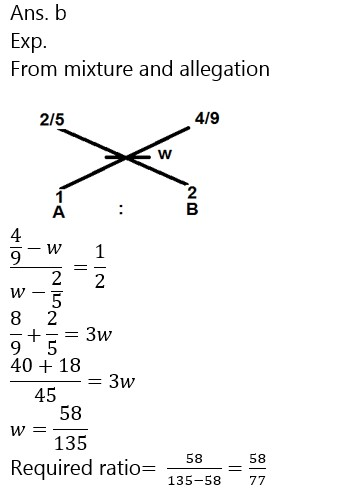1. A and B together can complete a work in 12 days. ‘A’ alone can complete in 20 days. If B does the work only half a day daily, then in how many days A and B together can complete the work?

(a) 14 days

(b) 15 days

(c)  50/3 days

(d) 18 days

(e) 9 days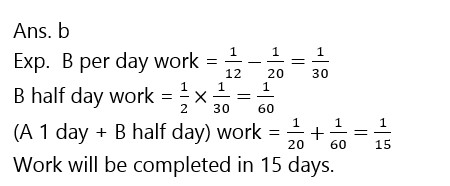1. A cylindrical tube open at both ends is made of metal. The internal diameter of the tube is 6 cm and length of the tube is 14 cm. If the thickness of the metal used is 1 cm, then the outer curved surface area of the tube is

(a)  326 cm^2

(b) 126 cm^2

(c) 152 〖cm〗^2

(d) 616 cm^2

(e) 352 cm^2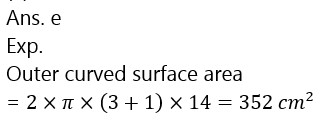1. Two numbers are in the ratio of 3 : 1. If the larger number is 12 more than the smaller number, find the smallest number that should be subtracted from the product of the numbers so that remainder is divisible by sum of the numbers.

(a) 12

(b) 18

(c) 8

(d) 15

(e) 61. When the price of tea was increased by 25%, a family reduced its consumption in such a way that expenditure on tea was only 20% more than before. If 25 kg were consumed per month before, find the new monthly consumption.

(a) 24 kg

(b) 20 kg

(c) 34 kg

(d) 40 kg

(e) 22 kg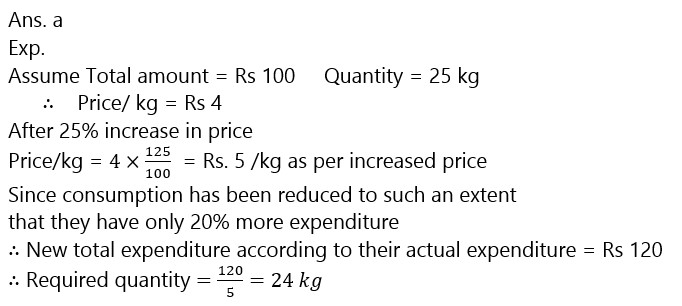### 2023 Preparation Kit PDF

#### Most important PDF’s for Bank, SSC, Railway and Other Government Exam : Download PDF Now

AATMA-NIRBHAR Series- Static GK/Awareness Practice Ebook PDF Get PDF here
The Banking Awareness 500 MCQs E-book| Bilingual (Hindi + English) Get PDF here
AATMA-NIRBHAR Series- Banking Awareness Practice Ebook PDF Get PDF here
Computer Awareness Capsule 2.O Get PDF here
AATMA-NIRBHAR Series Quantitative Aptitude Topic-Wise PDF 2020 Get PDF here
Memory Based Puzzle E-book | 2016-19 Exams Covered Get PDF here
Caselet Data Interpretation 200 Questions Get PDF here
Puzzle & Seating Arrangement E-Book for BANK PO MAINS (Vol-1) Get PDF here
ARITHMETIC DATA INTERPRETATION 2.O E-book Get PDF here

3